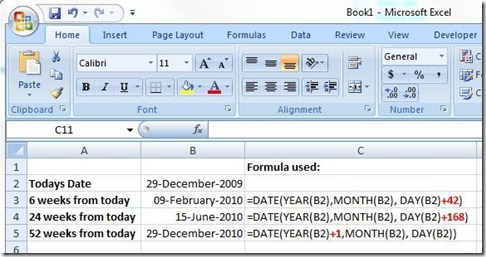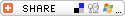## Excel: how do I calculate the date 24 weeks from today?

The question was:

Need to create series with dates in intervals of 6 weeks 24 weeks and 52 weeks. want it to be set to do it automatically. Can anyone help me?

The answer is simple.  You can use the Excel Date functions to do this:To break down what is happening here, using “24 weeks from today”, as an example.

The formula is ` =DATE(YEAR(B2),MONTH(B2), DAY(B2)+168) =DATE(2009,MONTH(B2), DAY(B2)+168) =DATE(2009,12, DAY(B2)+168) =DATE(2009,12,29+168)` <- (168 days equals 7 days time 24 weeks) ` =15 June 2010`

Update:
Another way to do this is by using the TODAY verb.
ie.  `=TODAY()+168`
(with thanks to The Angry Technician)